### Linear Maps Between Vector Spaces: Higher Order Tensors

### First and Second Order Tensors in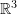For the discussion in this section, we will assume a right handed orthonormal basis set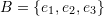and an alternate right handed orthonormal basis set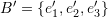. The two basis sets are related by the matrix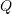whose components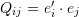.
If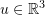has components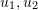and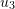in the basis set set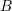, and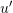is the representation ofin the basis set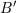with components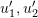and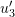, then, these components are related with the relationship:

(1)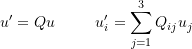Similarly, consider the tensor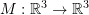. If its matrix representation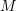has components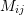whenis chosen as the basis set for, and if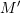is the matrix representation with components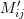whenis chosen as the basis set for, then, these components are related with the relationship:

(2)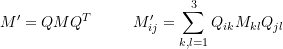Vectors inhave 3 independent components and are called first order tensors. Linear operators from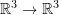have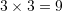independent components and are termed second order tensors.

### Third Order Tensors inThe linear map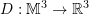where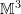is the set of all linear operatorsis called a third order tensor.
In the following we will show how the components of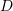change when the orthonormal basis set for the underlying spaceis changed.
The matrix representation ofwhenis chosen as the basis set forhas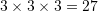independent components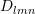such that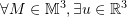such that:

(3)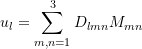Similarly, Ifis chosen as the basis set, then the components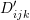would relate the components ofandas follows:

(4)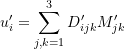However, premultiplying (3) with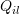and summing overproduces:

(5)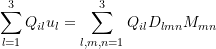By replacing the components ofin (5) with the inverse of the relationship (2) and using (1) we get:

(6)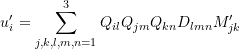Comparing (4) and (6), the componentsare related to the componentsby the following relationship:

(7)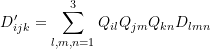(7) could have also been obtained by noticing the following: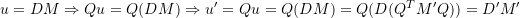From which the relationship between the components ofand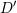can be obtained.

### Fourth Order Tensors inThe linear map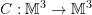whereis the set of all linear operatorsis called a fourth order tensor.
In the following we will show how the components of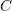change when the orthonormal basis set for the underlying spaceis changed.
The matrix representation ofwhenis chosen as the basis set forhas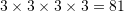independent components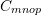such that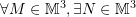such that:

(8)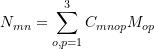Similarly, Ifis chosen as the basis set, then the components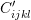would relate the components ofand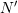as follows:

(9)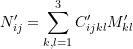However, premultiplying (8) with the components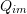and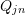and summing overandresults in:

(10)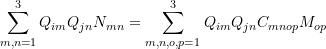By replacing the components ofin (10) with the inverse of the relationship (2) and using (2) we get:

(11)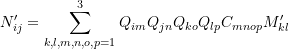Comparing (11) and (9), the componentsare related to the componentsby the following relationship:

(12)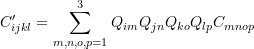(12) could have also be obtained by noticing the following: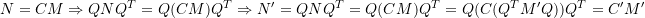From which the relationship between the components ofandcan be obtained.